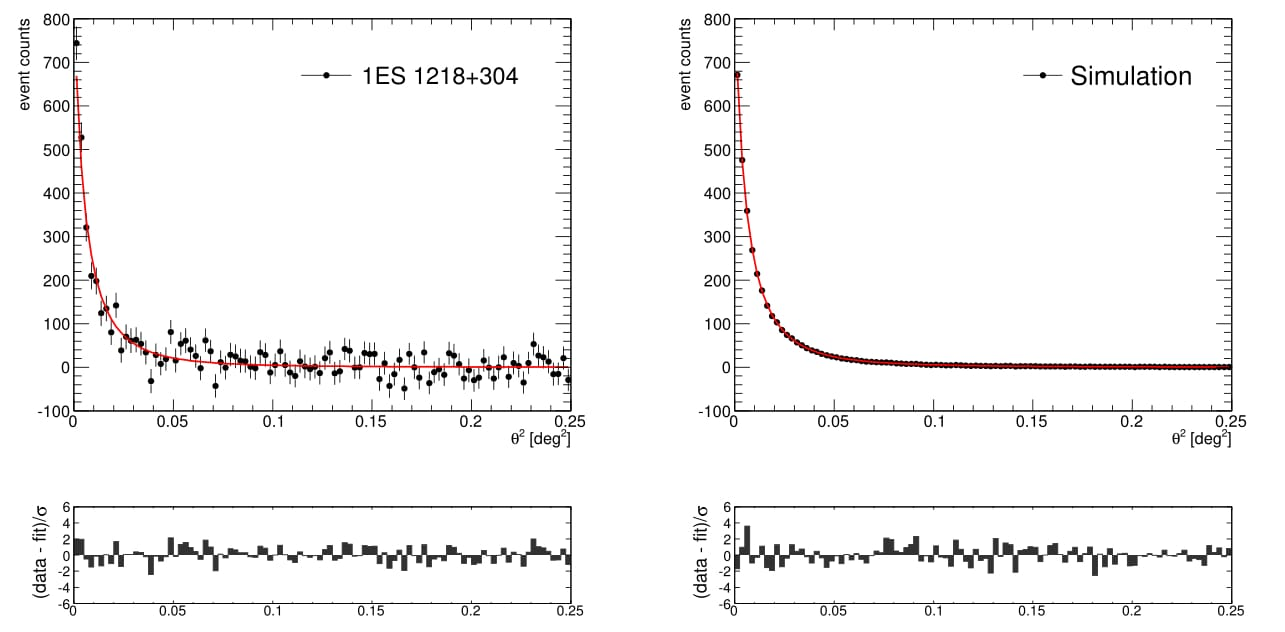Fitted θ2 distribution for 1ES 1218+304 and its simulated counterpart.

#### Contacts: Elisa Pueschel

The intergalactic magnetic field (IGMF) is known to be very weak, but the exact strength and correlation length are not known. Measuring these parameters would allow discrimination between competing theories of the field's generation. In this paper, constraints are placed on the IGMF strength via a search for angularly-broadened cascade emission from blazars. Cascade emission is produced when multi-TeV photons from blazars interact with extragalactic background light photons to produce electron-positron pairs, which then interact with cosmic microwave background photons via inverse-Compton scattering to produce gamma-rays. The IGMF deflects the electrons and positrons, leading to an angular broadening and time delay of the cascade component with respect to the unscattered primary emission.

No evidence of angular extension beyond the VERITAS point spread function was found in a study of seven blazars: Mrk 421, Mrk 501, VER J0521+211, H 1426+428, 1ES 0229+200, 1ES 1218+304, and PG 1553+113. The predicted cascade fraction, based on the source's redshift and spectral properties, is greatest for 1ES 1218+304. Based on the non-detection of an angular extension from 1ES 1218+304, an IGMF strength of around 10-14 G is excluded at the 95% confidence level. The exclusion range is found to vary significantly depending on assumptions about the source's intrinsic energy spectrum. Upper
limits on the flux due to extended emission are set for all sources.

FITS files: N/A

Figures from paper (click to get full size image):

Figure 1:  Comparison between the angular profiles of Mrk 501 and 1ES 1218+304 and their simulated counterparts. The results of a Χ 2 probability test are shown in Table 1 for all sources.
Figure 2: Fitted θ2 distribution for 1ES 1218+304 and its simulated counterpart.

Figure 3: The left panel shows the dependence of the width of the simulated angular distribution on the cascade fraction fc for 1ES 1218+304. This is compared against the width of the angular distribution measured in data, wdata.

Figure 4:  The 95% CL upper limits on the cascade fraction fc as a function of IGMF strength, for different assumptions about the intrinsic spectrum of 1ES 1218+304 and for two different EBL models.

Figure 5: Comparison between the angular profiles of the observed sources and their simulated counterparts. The results of a Χ 2 probability test are shown in Table 1.

Figure 6: Fitted θ 2 distribution for Mrk 421 and its simulated counterpart.

Figure 7: Fitted Θ2 distribution for Mrk 501 and its simulated counterpart.

Figure 8: Fitted Θ2 distribution for VER J0521+211 and its simulated counterpart.

Figure 9: Fitted Θ2 distribution for H 1426+428 and its simulated counterpart.

Figure 10: Fitted Θ 2 distribution for 1ES 0229+200 and its simulated counterpart.

Figure 11: Fitted Θ2 distribution for PG 1553+113 and its simulated counterpart.